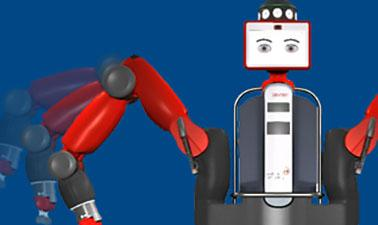# Robotics: Kinematics and Mathematical Foundations

Master the foundational math concepts that drive robotics and put them into practice using MATLAB.This course is archived
Estimated 12 weeks
8–10 hours per week
Self-paced

Welcome to the first course in the Robotics MicroMasters series. This is an advanced course designed for learners who have a bachelor's degree in engineering or a similar field.

Learners will succeed in this course if they have familiarity with basic operations on matrices and vectors, as well as exposure to derivatives and partial derivatives.

The fundamental challenge this course addresses is how one can create robots that operate well in the real world.

### At a glance

• Institution: PennX
• Subject: Computer Science
• Prerequisites:
• College-level algebra and trigonometry (matrices, vectors)
• Fundamentals of calculus (derivatives, partial derivatives)
• Basic knowledge of computer programming (variables, functions, control flow, some knowledge of graphs)
• Language: English

# What you'll learn

Skip What you'll learn
• How to describe, analyze and think critically about fundamental problems in robotics, such as how to change the position or configuration of a robot
• The role of mathematics in describing robotic arms, mobile robots and other robotic platforms
• How to use the industry standard programming environment, MATLAB

# Syllabus

Skip Syllabus

Math Fundamentals
Week 1: Vector spaces, inner products, vector norms, orthogonality
Week 2: Linear transformations, matrix multiplication, matrix groups
Week 3: Coordinate transformations, rigid transformations, rotation matrices quaternions, Matrix groups SE(2) and SE(3)
Week 4: Project

Robot Kinematics
Week 5: Kinematic chains, forward kinematics,
Week 6: Inverse kinematics
Week 7: Parallel mechanisms
Week 8: Project

_ Kinematic Path Planning _
Week 9: Graph based methods, Dijkstra’s method, A*Star
Week 10: RRT, configuration space
Week 11: Artificial potential fields
Week 12: Project